Angles

In the triangle ABC, the ratio of angles is: a:b = 4: 5. The angle c is 36°. How big are the angles a, b?

Result

a =  64
b =  80

Solution:

a=4/5*b
a+b+36 = 180

5a-4b = 0
a+b = 144

a = 64
b = 80

Calculated by our linear equations calculator.

Leave us a comment of example and its solution (i.e. if it is still somewhat unclear...):Be the first to comment!Next similar examples:

1. A triangleA triangle has an angle that is 63.1 other 2 are in ratio of 2:5 What are the measurements of the two angles?
2. Angles ratioThe internal angles of a triangle are in ratio 1:4:5 What kind of triangle is it? (solve internal angles and write down and discuss)
3. Isosceles triangleWhat are the angles of an isosceles triangle ABC if its base is long a=5 m and has an arm b=4 m.
4. Acute angles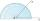Sizes of acute angles in the right-angled triangle are in the ratio 1: 3. What is size of the larger of them?
5. Triangle anglesThe angles α, β, γ in triangle ABC are in the ratio 6:2:6. Calculate size of angles.
6. Smallest internal angle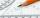Calculate what size has the smallest internal angle of the triangle if values of angles α:β:γ = 3:4:8
7. Angles 1It is true neighboring angles have not common arm?
8. Type of triangle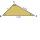How do I find the triangle type if the angle ratio is 2:3:7 ?
9. MedianThe median of the triangle LMN is away from vertex N 84 cm. Calculate the length of the median, which start at N.
10. Chord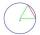Point on the circle is the end point of diameter and end point of chord length of radius. What angle between chord and diameter?
11. Angle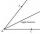Draw angle |∠ ABC| = 130° and built its axis. What angle is between axis angle and arm of angle?
12. DiagonalCan a rhombus have the same length diagonal and side?
13. Minute angle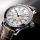Determine size of angle, which takes minute hand for 75 minutes.
14. TriangleProve whether you can construct a triangle ABC, if a=9 cm, b=10 cm, c=4 cm.
15. Center traverseIt is true that the middle traverse bisects the triangle?
16. Train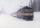The train passes part of the line for 95 minutes at speed 75 km/h. What speed would have to go in order to shorten the driving time of 20 minutes?
17. Obtuse angleWhich obtuse angle is creating clocks at 17:00?